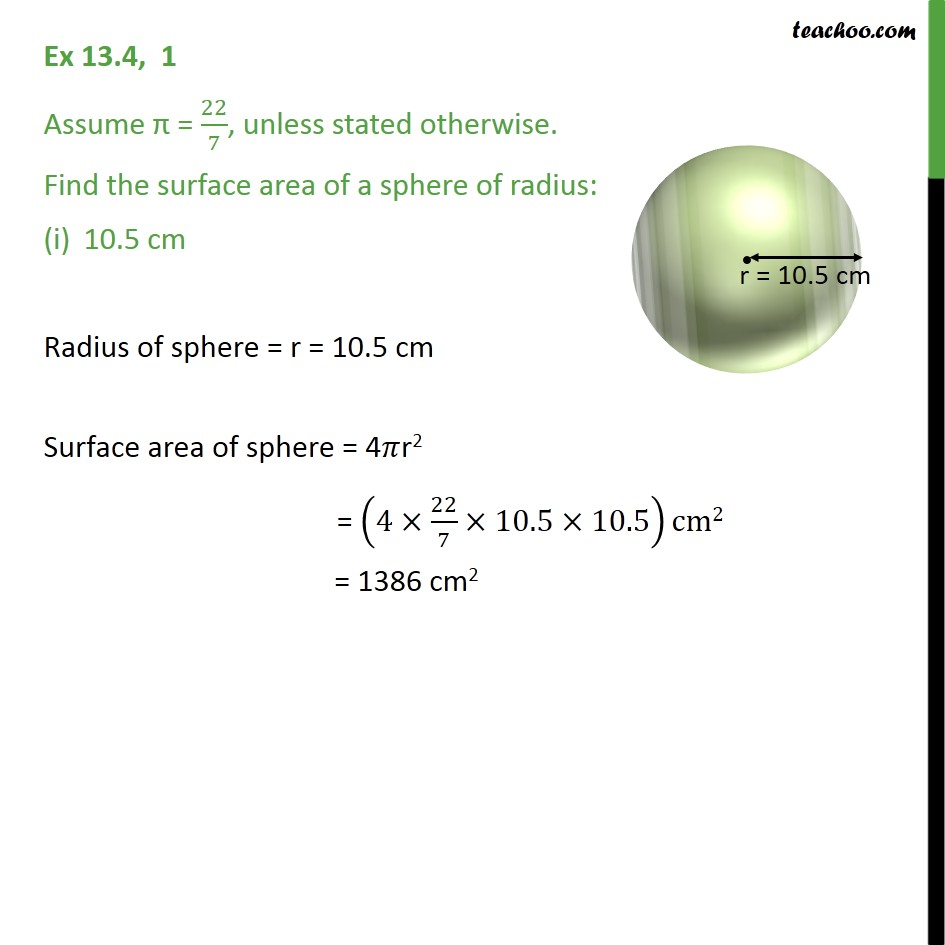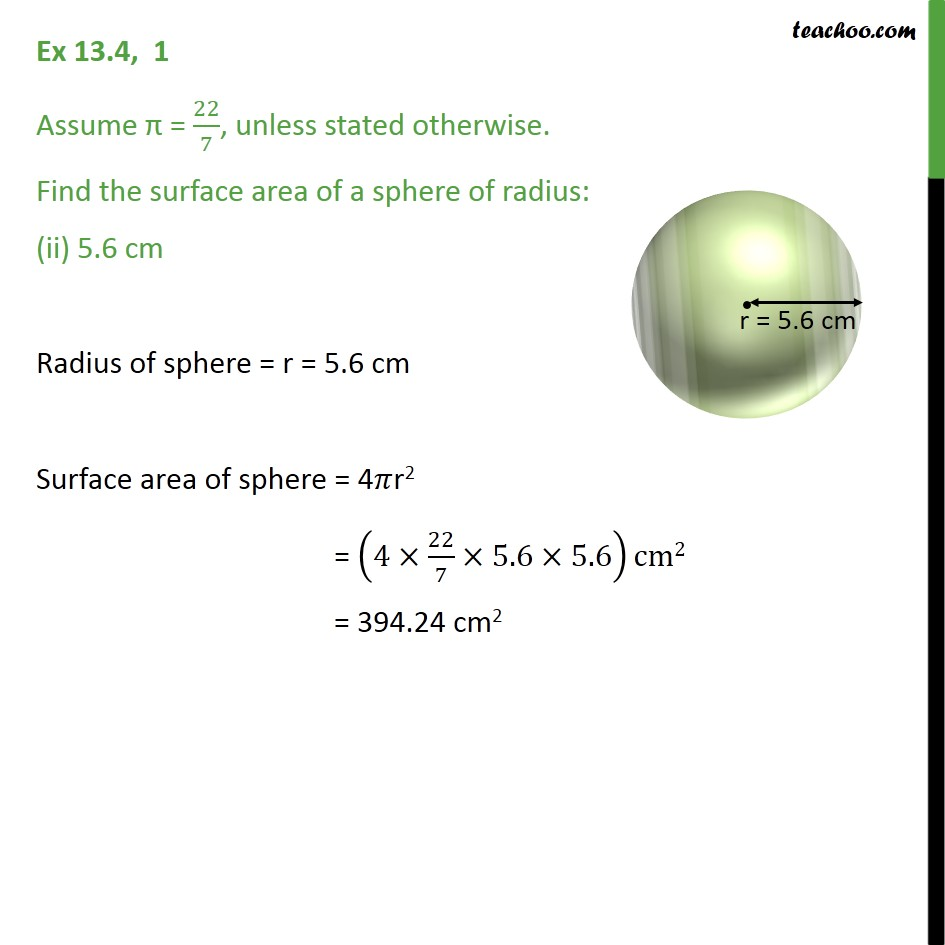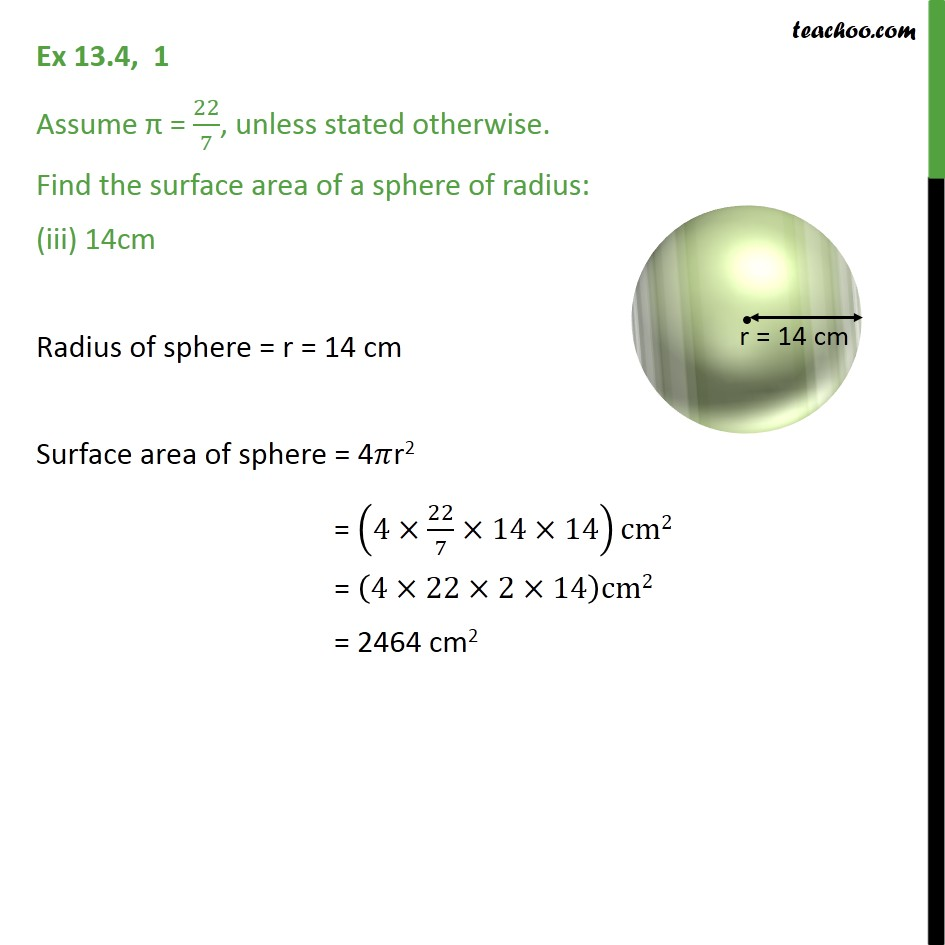Subscribe to our Youtube Channel - https://you.tube/teachoo

1. Chapter 13 Class 9 Surface Areas and Volumes
2. Serial order wise
3. Ex 13.4

Transcript

Ex 13.4, 1 Assume = 22/7, unless stated otherwise. Find the surface area of a sphere of radius: 10.5 cm Radius of sphere = r = 10.5 cm Surface area of sphere = 4 r2 = (4 22/7 10.5 10.5)cm2 = 1386 cm2 Ex 13.4, 1 Assume = 22/7, unless stated otherwise. Find the surface area of a sphere of radius: (ii) 5.6 cm Radius of sphere = r = 5.6 cm Surface area of sphere = 4 r2 = (4 22/7 5.6 5.6)cm2 = 394.24 cm2 Ex 13.4, 1 Assume = 22/7, unless stated otherwise. Find the surface area of a sphere of radius: (iii) 14cm Radius of sphere = r = 14 cm Surface area of sphere = 4 r2 = (4 22/7 14 14)cm2 = (4 22 2 14)cm2 = 2464 cm2

Ex 13.4